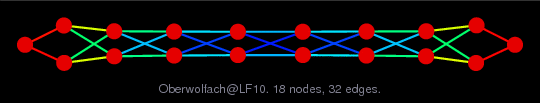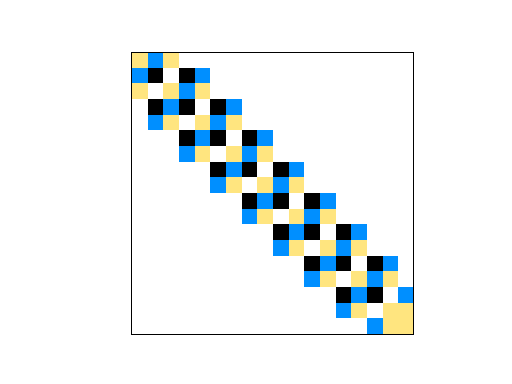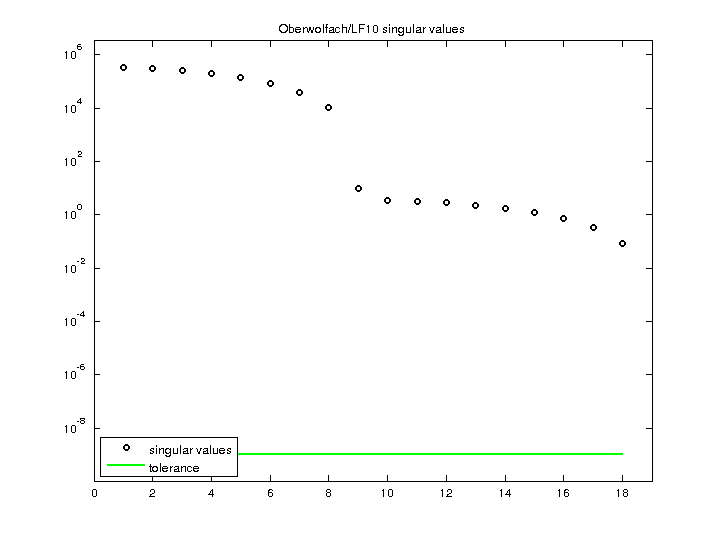Matrix: Oberwolfach/LF10

Description: Oberwolfach: linear 1D beam(undirected graph drawing)• Matrix group: Oberwolfach
• download as a MATLAB mat-file, file size: 928 bytes. Use UFget(1438) or UFget('Oberwolfach/LF10') in MATLAB.

 Matrix properties number of rows 18 number of columns 18 nonzeros 82 structural full rank? yes structural rank 18 # of blocks from dmperm 1 # strongly connected comp. 1 explicit zero entries 0 nonzero pattern symmetry symmetric numeric value symmetry symmetric type real structure symmetric Cholesky candidate? yes positive definite? yes

 author J. Lienemann, A. Greiner, J. Korvink editor E. Rudnyi date 2004 kind model reduction problem 2D/3D problem? yes

 Additional fields size and type M sparse 18-by-18 E sparse 18-by-18 B sparse 18-by-1 C sparse 1-by-18

Notes:

```Primary matrix in this model reduction problem is the Oberwolfach K matrix
```

 Ordering statistics: result nnz(chol(P*(A+A'+s*I)*P')) with AMD 58 Cholesky flop count 2.0e+02 nnz(L+U), no partial pivoting, with AMD 98 nnz(V) for QR, upper bound nnz(L) for LU, with COLAMD 58 nnz(R) for QR, upper bound nnz(U) for LU, with COLAMD 86

 SVD-based statistics: norm(A) 333192 min(svd(A)) 0.0864259 cond(A) 3.85524e+06 rank(A) 18 sprank(A)-rank(A) 0 null space dimension 0 full numerical rank? yes

 singular values (MAT file): click here SVD method used: s = svd (full (A)) status: ok